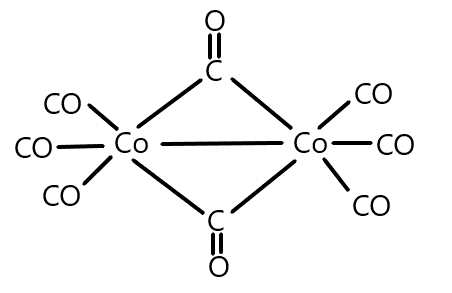# What is the oxidation state of Cobalt in the following molecule?A) $3$B) $1$C) $2$D) $0$Verified
Now, in the given molecule, we can see that the ligand present is the Carbonyl group which we know that is a neutral ligand and thus possesses zero charge. A total of eight carbonyl groups are present as ligands which will contribute a zero charge on the central atom that is cobalt because it is a homoleptic complex and no other ligand is present. And we can write the complex as: $[Co{(CO)_8}]$
Note: Always remember that carbonyl and cobalt seem similar but carbonyl contains $CO$ both in capital and it is a neutral ligand whereas $Co$ is a metal atom Cobalt. A neutral ligand is the one which does not contain any charge and electron pairs to donate. Along with $CO$, $N{H_3}$ and ${H_2}O$ are also neutral ligands. Halogens are considered anionic ligands and positively charged ligands are cationic ligands.